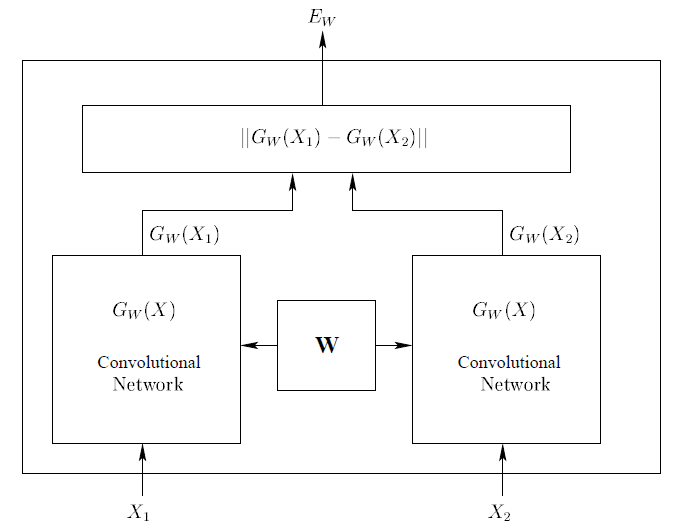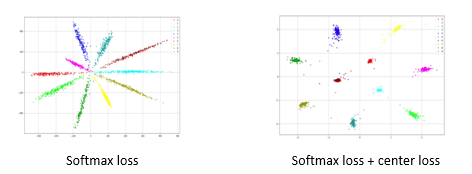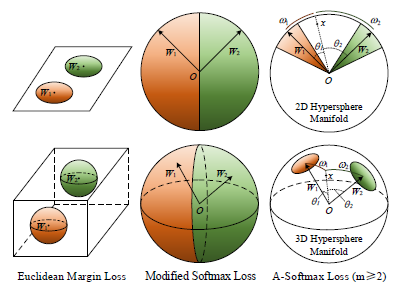简介Contrastive LossContrastive loss 定义如下：
$l(W)=∑_{i=1}^P L(W,(Y,X_1,X_2 )^i)$

$L(W,(Y,X_1,X_2 )^i )=(1-Y) L_G (E_W (X_1,X_2 )^i )+YL_I (E_W (X_1,X_2 )^i)$

$L(W,(Y,X_1,X_2 )^i )=(1-Y) E_W (X_1,X_2 )^i-Y(E_W (X_1,X_2 )^i))$

Triplet Loss

FaceNet  文中提出了Triplet Loss，与Contrastive loss的输入二元组不同的是，Triplet输入时人脸三元组。随机从某个人照片中选择一张，叫做Anchor；再从这个人照片中选择另一个照片，叫做Positive；最后随机选择一张其他人的照片，叫做Negative， 这样就构成了图像的三元组。图5就是三元组的一个例子。$L= ∑_i^N [∥f(x_i^a )-f(x_i^p )∥_2^2-∥f(x_i^a )-f(x_i^n )∥_2^2+α]_+$

Center Loss

$L_C= \frac{1}{2} ∑_{i=1}^m (∥x_i-c_{y_i}∥)_2^2$

$C_{yi} \in R^d$表示第$Y_i$ 类的特征中心。Center loss目标是让每类的特征尽可能集中，让每类在特征空间内所占的范围尽可能小，测试时当新的类别出现时，不会误判为已有的类别。结合center loss和softmax loss在一起，在Mnist的结果如图6右图所示，每类所占的空间极小。新的类出现，减少了误判的可能。A-Softmax Loss

$W_1^Tx+b = X_2^Tx+b \to (W_1^T - W_2^T)x - (b_1 - b_2) = 0$

$||W_1||||x||cos(\theta_1) + b_1 = ||W_2||||x||cos(\theta_2) + b_2$

$L_i=\frac{1}{N} ∑_i-\log(\frac{ e^{∥x_i∥\cos⁡(mθ_(y_i ),i) }}{e^{∥xi∥\cos(mθyi,i)} + \sum_{j ≠yi}e^{∥xi∥\cos(θj,i)}})$

Softmax，Modified Softmax， A-Softmax作用下的特征分布如图7所示：Cosine 距离是一个非线性距离，在人脸度量上比线性的欧式距离更有优势，加入超参数m，相当于在原有角度距离的基础上，限制了类内距离，保证类间距离大的同时类内距离小。正是如此，该算法也取得了Megaface竞赛的第一名。

参考文献：

 Alex Krizhevsky, Ilya Sutskever, Geoffrey E. Hinton ImageNet Classification with Deep Convolutional Neural Networks NIPS 2012
 http://vis-www.cs.umass.edu/lfw/
 http://www.cbsr.ia.ac.cn/english/CASIA-WebFace-Database.html
 https://www.microsoft.com/en-us/research/project/ms-celeb-1m-challenge-recognizing-one-million-celebrities-real-world/
 Sumit Chopra, Raia Hadsell, Yann LeCun Learning a Similarity Metric Discriminatively, with Application to Face Verification. CVPR 2005
 Florian Schroff, Dmitry Kalenichenko , James Philbin. FaceNet: A Unified Embedding for Face Recognition and Clustering CVPR 2015
 Yandong Wen, Kaipeng Zhang, Zhifeng Li, Yu Qiao. A Discriminative Feature Learning Approach
for Deep Face Recognition ECCV 2016
 Weiyang Liu, Yandong Wen, Zhiding Yu, Ming Li, Bhiksha Raj, Le Song SphereFace: Deep Hypersphere Embedding for Face Recognition CVPR 2017